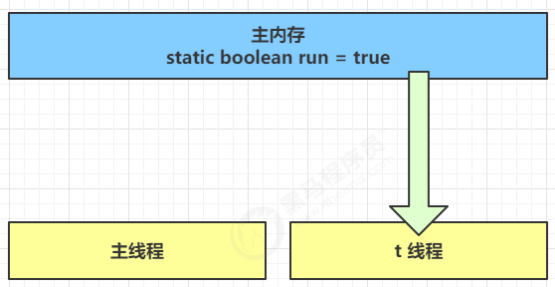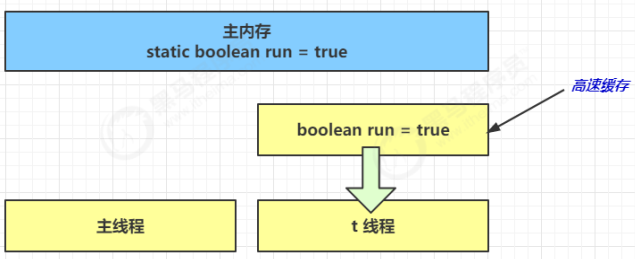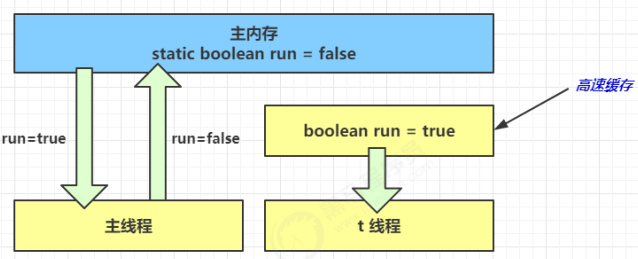### 8，Java 内存模型

JMM（Java Memory Model） 体现在以下几个方面：

• 原子性：保证指令不会受到线程上下文切换的影响
• 使用 synchronized
• 可见性：保证指令不会受 cpu 缓存的影响
• 使用 volatile（比较轻量） 或 synchronized（比较重量）
• 有序性：保证指令不会受 cpu 指令并行优化的影响
• volatile 可以禁止指令重排
• synchronized 也可以禁止指令重排
• volatile 关键字可以禁止该关键字修饰的变量，变量代码出现的地方之前的代码发生指令重排
volatile boolean ready = false;

/////////////////////////
num = 2;


volatile 的原理：其底层实现原理是内存屏障

• 对 volatile 变量的写指令后会加入写屏障
• 对 volatile 变量的读指令后会加入读屏障

#### 1，可见性问题 volatile

// 退不出的循环
// main 线程对 run 变量的修改对于 t 线程不可见，导致了 t 线程无法停止
static boolean run = true;

public static void main(String[] args) throws InterruptedException {
while(run){
// ....
}
});

t.start();
sleep(1);
run = false; // 线程t不会如预想的停下来
}


• 初始状态， t 线程刚开始从主内存读取了 run 的值到工作内存
•• 因为 t 线程要频繁从主内存中读取 run 的值，JIT 编译器会将 run 的值缓存至自己工作内存中的高速缓存中， 减少对主存中 run 的访问，提高效率
•• 1 秒之后，main 线程修改了 run 的值，并同步至主存，而 t 是从自己工作内存中的高速缓存中读取这个变量 的值，结果永远是旧值
•• volatile 它可以用来修饰成员变量和静态成员变量，可以避免线程从自己的工作缓存中查找变量的值，必须到主存中获取它的值，线程操作 volatile 变量都是直接操作主存
• 它保证的是在多个线程之间，一个线程对 volatile 变量的修改对另一个线程可见， 不能保证原子性，仅用在一个写线程，多个读线程的情况
• 注意 synchronized 语句块既可以保证代码块的原子性，也同时保证代码块内变量的可见性。但缺点是synchronized 是属于重量级操作，性能相对更低

#### 2，有序性问题-指令重排

JVM 会在不影响正确性的前提下，可以调整语句的执行顺序，比如下面代码：

static int i;
static int j;
// 在某个线程内执行如下赋值操作
i = ...;
j = ...;


i = ...;
j = ...;


j = ...;
i = ...;


int num = 0;

// 线程1 执行此方法
public void actor1(I_Result r) {
r.r1 = num + num;
} else {
r.r1 = 1;
}
}

// 线程2 执行此方法
public void actor2(I_Result r) {
// 注意这两处代码可能发生指令重排
num = 2;
}


I_Result 是一个对象，有一个属性 r1 用来保存结果，问，可能的结果有几种？

• 线程1 先执行，这时 ready = false，所以进入 else 分支结果为 1
• 线程2 先执行 num = 2，但没来得及执行 ready = true，线程1 执行，还是进入 else 分支，结果为1
• 线程2 执行到 ready = true，线程1 执行，这回进入 if 分支，结果为 4

num = 2;


ready = true;
num = 2;


volatile boolean ready = false;


num = 2;


#### 3，volatile 原理

volatile 可以解决可见性问题和指令重排问题。

volatile 的其底层实现原理是内存屏障

• 对 volatile 变量的写指令后会加入写屏障
• 对 volatile 变量的读指令后会加入读屏障

volatile 对可见性问题的处理：

public void actor2(I_Result r) {
num = 2;
// 写屏障位置
}


public void actor1(I_Result r) {
// 读屏障位置
r.r1 = num + num;
} else {
r.r1 = 1;
}
}


volatile 对指令重排问题的处理：

public void actor2(I_Result r) {
num = 2;
// 写屏障位置
}


public void actor1(I_Result r) {
// 读屏障位置
r.r1 = num + num;
} else {
r.r1 = 1;
}
}


#### 4，happens-before 规则

happens-before 规定了对共享变量的写操作对其它线程的读操作可见，它是可见性与有序性的一套规则总结，抛开以下 happens-before 规则，JMM 并不能保证一个线程对共享变量的写，对于其它线程对该共享变量的读可见。

• 线程解锁 m 之前对变量的写，对于接下来对 m 加锁的其它线程对该变量的读可见
static int x;
static Object m = new Object();

synchronized(m) {
x = 10;
}
},"t1").start();

synchronized(m) {
System.out.println(x);
}
},"t2").start();

• 线程对 volatile 变量的写，对接下来其它线程对该变量的读可见
volatile static int x;

x = 10;
},"t1").start();

System.out.println(x);
},"t2").start();

• 线程 start 前对变量的写，对该线程开始后对该变量的读可见
static int x;

x = 10;

System.out.println(x);
},"t2").start();

• 线程结束前对变量的写，对其它线程得知它结束后的读可见（比如其它线程调用 t1.isAlive() 或 t1.join()等待它结束）
static int x;

x = 10;
},"t1");

t1.start();
t1.join();

System.out.println(x);

• 线程 t1 打断 t2（interrupt）前对变量的写，对于其他线程得知 t2 被打断后对变量的读可见（通过t2.interrupted 或 t2.isInterrupted）
static int x;

public static void main(String[] args) {

while(true) {
System.out.println(x);
break;
}
}
},"t2");

t2.start();

sleep(1);
x = 10;
t2.interrupt();
},"t1").start();

while(!t2.isInterrupted()) {
}

System.out.println(x);
}

• 对变量默认值（0，false，null）的写，对其它线程对该变量的读可见
• 具有传递性，如果 x hb-> y 并且 y hb-> z 那么有 x hb-> z ，配合 volatile 的防指令重排，有下面的例子
volatile static int x;
static int y;

y = 10;
x = 20;
},"t1").start();

// x=20 对 t2 可见, 同时 y=10 也对 t2 可见
System.out.println(x);
},"t2").start();


### 9，共享模型之无锁

#### 1，问题：实现一个多线程取款

Account 接口：

public interface Account {

// 获取余额
Integer getBalance();

// 取款
void withdraw(Integer amount);

/**
* 方法内会启动 1000 个线程，每个线程做 -10 元 的操作
* 如果初始余额为 10000 那么正确的结果应当是 0
*/
static void demo(Account account) {
long start = System.nanoTime();

for (int i = 0; i < 1000; i++) {
account.withdraw(10);
}));
}

ts.forEach(t -> {
try {
t.join();
} catch (InterruptedException e) {
e.printStackTrace();
}
});

long end = System.nanoTime();
System.out.println(account.getBalance()
+ " cost: " + (end-start)/1000_000 + " ms");
}
}


#### 2，使用锁实现 synchronized

public class AccountSynchronized implements Account {
private Integer balance;

public AccountSynchronized(Integer balance) {
this.balance = balance;
}

@Override
public synchronized Integer getBalance() {
return balance;
}

@Override
public synchronized void withdraw(Integer amount) {
balance -= amount;
}

public static void main(String[] args) {
Account a = new AccountSynchronized(10000);
Account.demo(a);
}
}


#### 3，使用无锁实现 Atomic

public class AccountAtomic implements Account {

private AtomicInteger balance;

public AccountAtomic(Integer balance) {
this.balance = new AtomicInteger(balance);
}

@Override
public Integer getBalance() {
return balance.get();
}

@Override
public void withdraw(nteger amount) {
while (true) {
int prev = balance.get();
int next = prev - amount;

// compareAndSet 是原子操作
// 先比较再赋值
if (balance.compareAndSet(prev, next)) {
break;
}
}

// 可以简化为下面的方法
}

public static void main(String[] args) {
Account a = new AccountAtomic(10000);
Account.demo(a);
}
}


• 无锁情况下，即使重试失败，线程始终在高速运行，没有停歇，而 synchronized 会让线程在没有获得锁的时候，发生上下文切换，进入阻塞
• 线程就好像高速跑道上的赛车，高速运行时，速度超快，一旦发生上下文切换，就好比赛车要减速、熄火，等被唤醒又得重新打火、启动、加速… 恢复到高速运行，代价比较大
• 单CPU无锁情况下，因为线程要保持运行，需要额外 CPU 的支持，CPU 在这里就好比高速跑道，没有额外的跑道，线程想高速运行也无从谈起，虽然不会进入阻塞，但由于没有分到时间片，仍然会进入可运行状态，还是会导致上下文切换

#### 4，CAS 的特点

• CAS 是基于乐观锁的思想：最乐观的估计，不怕别的线程来修改共享变量，就算改了也没关系，我吃亏点再重试呗
• synchronized 是基于悲观锁的思想：最悲观的估计，得防着其它线程来修改共享变量，我上了锁你们都别想改，我改完了解开锁，你们才有机会
• CAS 体现的是无锁并发、无阻塞并发，请仔细体会这两句话的意思
• 因为没有使用 synchronized，所以线程不会陷入阻塞，这是效率提升的因素之一
• 如果竞争激烈，可以想到重试必然频繁发生，反而效率会受影响

### 10，原子包装类

#### 1，原子整数类

java.util.concurrent.atomic 包中提供了 3 个原子整数类：

• AtomicBoolean
• AtomicInteger
• AtomicLong

AtomicInteger i = new AtomicInteger(0);

// 获取并自增（i = 0, 结果 i = 1, 返回 0），类似于 i++
System.out.println(i.getAndIncrement());

// 自增并获取（i = 1, 结果 i = 2, 返回 2），类似于 ++i
System.out.println(i.incrementAndGet());

// 自减并获取（i = 2, 结果 i = 1, 返回 1），类似于 --i
System.out.println(i.decrementAndGet());

// 获取并自减（i = 1, 结果 i = 0, 返回 1），类似于 i--
System.out.println(i.getAndDecrement());

// 获取并加值（i = 0, 结果 i = 5, 返回 0）

// 加值并获取（i = 5, 结果 i = 0, 返回 0）

// 获取并更新（i = 0, p 为 i 的当前值, 结果 i = -2, 返回 0）
// 其中函数中的操作能保证原子，但函数需要无副作用
System.out.println(i.getAndUpdate(p -> p - 2));

// 更新并获取（i = -2, p 为 i 的当前值, 结果 i = 0, 返回 0）
// 其中函数中的操作能保证原子，但函数需要无副作用
System.out.println(i.updateAndGet(p -> p + 2));

// 获取并计算（i = 0, p 为 i 的当前值, x 为参数1, 结果 i = 10, 返回 0）
// 其中函数中的操作能保证原子，但函数需要无副作用
// getAndUpdate 如果在 lambda 中引用了外部的局部变量，要保证该局部变量是 final 的
// getAndAccumulate 可以通过 参数1 来引用外部的局部变量，但因为其不在 lambda 中因此不必是 final
System.out.println(i.getAndAccumulate(10, (p, x) -> p + x));

// 计算并获取（i = 10, p 为 i 的当前值, x 为参数1, 结果 i = 0, 返回 0）
// 其中函数中的操作能保证原子，但函数需要无副作用
System.out.println(i.accumulateAndGet(-10, (p, x) -> p + x));


#### 2，原子引用类

• AtomicReference
• AtomicMarkableReference
• AtomicStampedReference
##### 1，AtomicReference
class DecimalAccountSafeCas implements DecimalAccount {
AtomicReference<BigDecimal> ref;	// 对 BigDecimal 类型 进行原子包装

public DecimalAccountSafeCas(BigDecimal balance) {
ref = new AtomicReference<>(balance);
}
}


ABA 问题示例：

static AtomicReference<String> ref = new AtomicReference<>("A");

public static void main(String[] args) throws InterruptedException {
log.debug("main start...");
// 获取值 A
// 这个共享变量被它线程修改过？
String prev = ref.get();
other();
sleep(1);
// 尝试改为 C
log.debug("change A->C {}", ref.compareAndSet(prev, "C"));
}

private static void other() {
log.debug("change A->B {}", ref.compareAndSet(ref.get(), "B"));
}, "t1").start();

sleep(0.5);

log.debug("change B->A {}", ref.compareAndSet(ref.get(), "A"));
}, "t2").start();
}


##### 2，AtomicStampedReference

static AtomicStampedReference<String> ref = new AtomicStampedReference<>("A", 0);

public static void main(String[] args) throws InterruptedException {
log.debug("main start...");
// 获取值 A
String prev = ref.getReference();
// 获取版本号
int stamp = ref.getStamp();
log.debug("版本 {}", stamp);
// 如果中间有其它线程干扰，发生了 ABA 现象
other();
sleep(1);
// 尝试改为 C，更新失败
log.debug("change A->C {}", ref.compareAndSet(prev, "C", stamp, stamp + 1));
}

private static void other() {
log.debug("change A->B {}", ref.compareAndSet(ref.getReference(), "B", ref.getStamp(), ref.getStamp() + 1));
log.debug("更新版本为 {}", ref.getStamp());
}, "t1").start();

sleep(0.5);

log.debug("change B->A {}", ref.compareAndSet(ref.getReference(), "A", ref.getStamp(), ref.getStamp() + 1));
log.debug("更新版本为 {}", ref.getStamp());
}, "t2").start();
}


AtomicStampedReference 可以给原子引用加上版本号，追踪原子引用整个的变化过程，如： A -> B -> A ->C ，通过AtomicStampedReference，我们可以知道，引用变量中途被更改了几次。

##### 3，AtomicMarkableReference

class GarbageBag {
String desc;

public GarbageBag(String desc) {
this.desc = desc;
}

public void setDesc(String desc) {
this.desc = desc;
}

@Override
public String toString() {
return super.toString() + " " + desc;
}
}

@Slf4j
public class TestABAAtomicMarkableReference {
public static void main(String[] args) throws InterruptedException {
GarbageBag bag = new GarbageBag("装满了垃圾");
// 参数2 mark 可以看作一个标记，表示垃圾袋满了
AtomicMarkableReference<GarbageBag> ref = new AtomicMarkableReference<>(bag, true);

log.debug("主线程 start...");
GarbageBag prev = ref.getReference();
log.debug(prev.toString());

log.debug("打扫卫生的线程 start...");
bag.setDesc("空垃圾袋");
while (!ref.compareAndSet(bag, bag, true, false)) {}
log.debug(bag.toString());
}).start();

log.debug("主线程想换一只新垃圾袋？");
boolean success = ref.compareAndSet(prev, new GarbageBag("空垃圾袋"), true, false);
log.debug("换了么？" + success);

log.debug(ref.getReference().toString());
}
}


#### 3，原子数组

• AtomicIntegerArray
• AtomicLongArray
• AtomicReferenceArray

#### 4，字段更新器

• AtomicReferenceFieldUpdater // 域 字段
• AtomicIntegerFieldUpdater
• AtomicLongFieldUpdater

### 11，共享模型之不可变类

#### 1，不可变类

SimpleDateFormat sdf = new SimpleDateFormat("yyyy-MM-dd");
for (int i = 0; i < 10; i++) {
try {
// sdf.parse 会出现问题，因为其不是线程安全的
log.debug("{}", sdf.parse("1951-04-21"));
} catch (Exception e) {
log.error("{}", e);
}
}).start();
}


SimpleDateFormat sdf = new SimpleDateFormat("yyyy-MM-dd");
for (int i = 0; i < 50; i++) {
synchronized (sdf) { // 同步处理
try {
log.debug("{}", sdf.parse("1951-04-21"));
} catch (Exception e) {
log.error("{}", e);
}
}
}).start();
}


DateTimeFormatter dtf = DateTimeFormatter.ofPattern("yyyy-MM-dd");
for (int i = 0; i < 10; i++) {
LocalDate date = dtf.parse("2018-10-01", LocalDate::from);
log.debug("{}", date);
}).start();
}


#### 2，不可变类的设计 final

final 的使用：

• 类和类中所有属性都是 final 的
• 类用 final 修饰保证了该类中的方法不能被覆盖，防止子类无意间破坏不可变性

• 通过创建副本对象来避免共享的手段称之为【保护性拷贝】

#### 3，不可变类与享元模式

JDK 中的享元模式：

• 在JDK中 Boolean，Byte，Short，Integer，Long，Character 等包装类提供了 valueOf 方法，例如 Long 的 valueOf 会缓存 -128~127 之间的 Long 对象，在这个范围之间会重用对象，大于这个范围，才会新建 Long 对象。
public static Long valueOf(long l) {
final int offset = 128;
if (l >= -128 && l <= 127) { // will cache
return LongCache.cache[(int)l + offset];
}
return new Long(l);
}


• Byte, Short, Long 缓存的范围都是 -128~127
• Character 缓存的范围是 0~127
• Integer的默认范围是 -128~127
• 最小值不能变
• 但最大值可以通过调整虚拟机参数 -Djava.lang.Integer.IntegerCache.high 来改变
• Boolean 缓存了 TRUE 和 FALSE